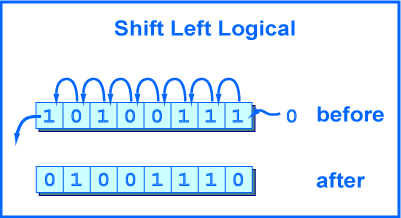2N, twice the original integer.

`0110 0001` = 9710.

`1100 0010` = 19410.

(However, if a 1-bit is shifted off the left side, then the number is ruined).

# Shift Left LogicalA shift left logical of one position moves each bit to the left by one. The low-order bit (the right-most bit) is replaced by a zero bit and the high-order bit (the left-most bit) is discarded.

Shifting by two positions is the same as performing a one-position shift two times. Shifting by zero positions leaves the pattern unchanged. Shifting an N-bit pattern left by N or more positions changes all of the bits to zero.

The picture shows the operation performed on eight bits. The original pattern is `1010 0111`. The resulting pattern is `0100 1110`.

The MIPS processor always performs the operation on a 32-bit register and puts the result in a 32-bit register.

```sll  d,s,shft      # \$d gets the bits in \$s
# shifted left logical
# by shft positions,
# where  0 ≤ shft < 32
```

The ALU (arithmetic/logic unit) which does the operation pays no attention to what the bits mean. If the bits represent an unsigned integer, then a left shift is equivalent to multiplying the integer by two.

### QUESTION 2:

Here is an 8-bit pattern. Shift it left (logical) by two. Then write the new 8-bit pattern as hex.

OriginalShift Left Two
0110 1111
0x6F